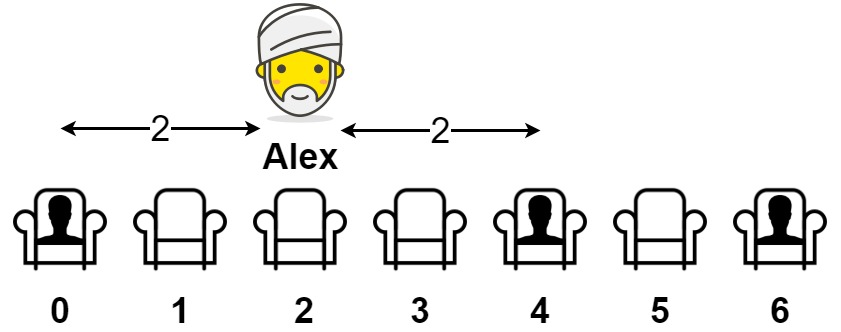## Algorithm

Problem Name: 849. Maximize Distance to Closest Person

You are given an array representing a row of `seats` where `seats[i] = 1` represents a person sitting in the `ith` seat, and `seats[i] = 0` represents that the `ith` seat is empty (0-indexed).

There is at least one empty seat, and at least one person sitting.

Alex wants to sit in the seat such that the distance between him and the closest person to him is maximized.

Return that maximum distance to the closest person.

Example 1:```Input: seats = [1,0,0,0,1,0,1]
Output: 2
Explanation:
If Alex sits in the second open seat (i.e. seats), then the closest person has distance 2.
If Alex sits in any other open seat, the closest person has distance 1.
Thus, the maximum distance to the closest person is 2.
```

Example 2:

```Input: seats = [1,0,0,0]
Output: 3
Explanation:
If Alex sits in the last seat (i.e. seats), the closest person is 3 seats away.
This is the maximum distance possible, so the answer is 3.
```

Example 3:

```Input: seats = [0,1]
Output: 1
```

Constraints:

• `2 <= seats.length <= 2 * 104`
• `seats[i]` is `0` or `1`.
• At least one seat is empty.
• At least one seat is occupied.

## Code Examples

### #1 Code Example with C++ Programming

```Code - C++ Programming```

``````
class Solution {
public:
int maxDistToClosest(vector& seats) {
int res = -1, d = 0;
for(auto x: seats) if(x) res = max(res, res == -1 ? d : d/2), d = 1; else d++;
return max(res, d - 1);
}
};
``````
Copy The Code &

Input

cmd
seats = [1,0,0,0,1,0,1]

Output

cmd
2

### #2 Code Example with Java Programming

```Code - Java Programming```

``````
class Solution {
public int maxDistToClosest(int[] seats) {
int n = seats.length;
int maxDistance = 0;
int start = -1;
for (int i = 0; i < n; i++) {
if (seats[i] == 1) {
maxDistance = start == -1 ? i : Math.max(maxDistance, (i - start) / 2);
start = i;
}
}
return Math.max(maxDistance, n - 1 - start);
}
}
``````
Copy The Code &

Input

cmd
seats = [1,0,0,0,1,0,1]

Output

cmd
2

### #3 Code Example with Javascript Programming

```Code - Javascript Programming```

``````const maxDistToClosest = function(seats) {
let left, right, res = 0, n = seats.length;
for (left = right = 0; right < n; ++right)
if (seats[right] === 1) {
if (left === 0) res = Math.max(res, right - left);
else res = Math.max(res, Math.floor((right - left + 1) / 2));
left = right + 1;
}
res = Math.max(res, n - left);
return res;
};
``````
Copy The Code &

Input

cmd
seats = [1,0,0,0]

Output

cmd
3

### #4 Code Example with Python Programming

```Code - Python Programming```

``````
class Solution:
def maxDistToClosest(self, seats):
d = {}
res = l = left = r = right = 0
for i, s in enumerate(seats):
if not s and left: d[i] = l = l + 1
elif s: l, left = 0, 1
for i in range(len(seats) - 1, -1, -1):
if not seats[i] and right and (i not in d or d[i] > r): d[i] = r = r + 1
elif seats[i]: r, right = 0, 1
return max(d.values())
``````
Copy The Code &

Input

cmd
seats = [1,0,0,0]

Output

cmd
3

### #5 Code Example with C# Programming

```Code - C# Programming```

``````
using System;

namespace LeetCode
{
public class _0849_MaximizeDistanceToClosestPerson
{
public int MaxDistToClosest(int[] seats)
{
int k = 0, n = seats.Length;
var result = 0;

for (int i = 0; i < n; i++)
{
if (seats[i] == 1)
k = 0;
else
{
k++;
result = Math.Max(result, (k + 1) / 2);
}
}

for (int i = 0; i < n; i++)
{
if (seats[i] == 1)
{
result = Math.Max(result, i);
break;
}
}

for (int i = n - 1; i >= 0; i--)
{
if (seats[i] == 1)
{
result = Math.Max(result, n - i - 1);
break;
}
}

return result;
}
}
}
``````
Copy The Code &

Input

cmd
seats = [0,1]

Output

cmd
1